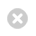Close

## UCL Mechanical Engineering Faculty of Engineering Sciences

Home# ENGF0003 Mathematical Modelling and Analysis 1

## ENGF0003 Mathematical Modelling and Analysis 1

This module is taught by the Faculty of Engineering Sciences as part of the Integrated Engineering Programme (IEP). Please consult the Integrated Engineering Programme website for the most up-to-date information about IEP modules.

FHEQ Level:
Term:
Term 1
UCL / ECTS Credits:
15 UCL / 7.5 ECTS
Previous Module Code:
ENGS103P
Prerequisites:
None

## Taught By

Dr Abel Nyamapfene (Module Coordinator):
Abel Nyamapfene

## Module Overview

This module has been especially designed to help students to develop an understanding of the mathematical concepts that underpin the study of engineering. In addition to learning new mathematical concepts, students will apply their newly acquired skills in solving practical engineering problems. They will do this by developing mathematical models of the engineering problems, and then simulating and analysing these models using software tools like MATLAB and Excel.
Students will use uses mathematical modelling and simulation techniques to integrate the acquisition and practice of mathematical concepts. This approach is underpinned by a suite of online mathematical support resources as well as a walk-in student-led Engineering Mathematics Support team.

## Topics Covered

• Introduction to mathematical modelling
• Describing the world
• Systems and signals
• Engineering systems modelling
• Probability and statistics

## Learning Outcomes

Upon completion of this module students will be able to:

• Recognise the connections between mathematics and engineering, and how mathematical ideas are embedded in engineering contexts.
• Represent real-world systems from engineering in a mathematical framework.
• Identify and draw upon a range of mathematical concepts, including Calculus, Linear Algebra and Differential Equations to analyse specific problems and identify the appropriate mathematics to realise a solution.
• Employ appropriate computer programming and modelling techniques and statistical analysis to efficiently solve and evaluate the performance of engineering systems.
• Use estimation, approximation and dimensional analysis to reduce complexity.
• Relate the behaviour of the output of mathematical models to the underlying physical or conceptual models of interest.
• Carry out engineering problem solving both collaboratively in a team and independently.
• Present and interpret mathematical results in effective and appropriate ways to varied audiences, including non-mathematical engineering audiences.

## Method of Instruction

This module is delivered through a blended learning approach integrating face-to-face lectures, weekly problem classes, structured online Moodle resources and mathematical
modelling and simulation tools like MATLAB and EXCEL.

## Assessment

This module is assessed through a combination of unseen written examination and coursework exercises.